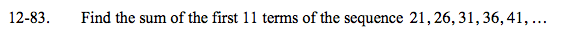### Home > A2C > Chapter 12 > Lesson 12.2.1 > Problem12-83

12-83.t(n) = 5n + 16

$S_{11}=\frac{n}{2}(t(1)+t(n))=\frac{11}{2}(21 + 71)$

S11 = 506